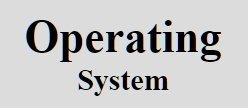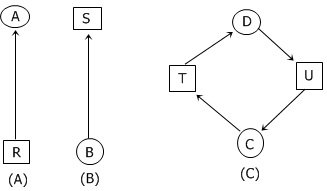A computer scientist/programmer named Holt showed how the four conditions for deadlock (as described in the previous tutorial) can be modelled using directed graphs. These graphs shown below.In the above resource allocation graphs, the figure A, B, and C represents:

Figure Represents
(A) Holding a resource
(B) Requesting a resource

According to Hold, these graphs have the following two types of nodes:

• Processes - shown in graph as circles
• Resources - shown in graph as squares

Here, in this graph, an arc from a square (resource node) to a circle (process node) means that the resource has previously been requested by, granted to, and is currently held by that process.

As you can see from the figure given above, resource R is currently assigned to process A in figure A.

An arc from a process to a resource means that the process is currently blocked waiting for that resource.

As you can see from the above figure, in the figure B, process B is waiting for resource S.

And, in the figure C, we see a deadlock, that is, the process C is waiting for the resource T, which is currently held by the process D. Here, process D is not about the release the resource T because it is waiting for resource U, held by C. Here both processes will wait forever.

Tools
Calculator